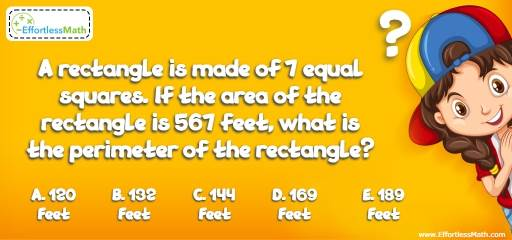# Geometry Puzzle – Challenge 63

This is another great puzzle to challenge and engage students in a fun way. Let's see who can solve this geometry-related challenge!## Challenge:

A rectangle is made of 7 equal squares. If the area of the rectangle is 567 feet, what is the perimeter of the rectangle?

A- 120 feet

B- 132 feet

C- 144 feet

D- 169 feet

E- 180 feet

### The Absolute Best Book to Challenge Your Smart Student!

The rectangle is made of 7 equal squares. Therefore, the ratio of the width and length of the rectangle is 1 to 7. Why?
Let X be the width of the rectangle. So,
$$X × 7X = 567 → 7X^2 = 567 → X^2 = 81 → X = 9$$
The width of the rectangle is 9 feet and the length is 63 feet.
(7 × 9 = 63)
The perimeter of the rectangle is:
2 × (9 + 63) = 144 feet

The Best Books to Ace Algebra

### What people say about "Geometry Puzzle – Challenge 63 - Effortless Math: We Help Students Learn to LOVE Mathematics"?

No one replied yet.

X
45% OFF

Limited time only!

Save Over 45%

SAVE $40 It was$89.99 now it is \$49.99